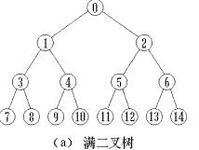1 #include<stdio.h>

2 #include<assert.h>

3

4 void binary_search(int arr[],int len,int num){

5         assert(arr);

6         int left = 0;

7         int right = len-1;

8         while(left<right){

9                 int mid = (left+right)/2;

10                 if(num == arr[mid]){

11                         printf("%d\n",mid);

12                         break;

13                 }

14                 else if(num > arr[mid])

15                         left = mid+1;

16                 else if(num < arr[mid])

17                         right = mid-1;

18                 else

19                         printf("not find\n");

20         }

21 }

22

23 int main(){

24         int arr[] = {1,2,3,4,5,6,7,8};

25         int len = sizeof(arr)/sizeof(arr);

26         int num = 7;

27         binary_search(arr,len,num);

28 return 0;

29 }

1.  while(left<right)里边界判断条件应该写成while(left<=right)，试想当数组只有一个元素时就会出现错误结果；

2. int mid = (left+right)/2;也有问题，当   left+right的值相当大超出了int型整数的范围时，就会溢出。应该写成 int mid = left+（right-left)/2 。